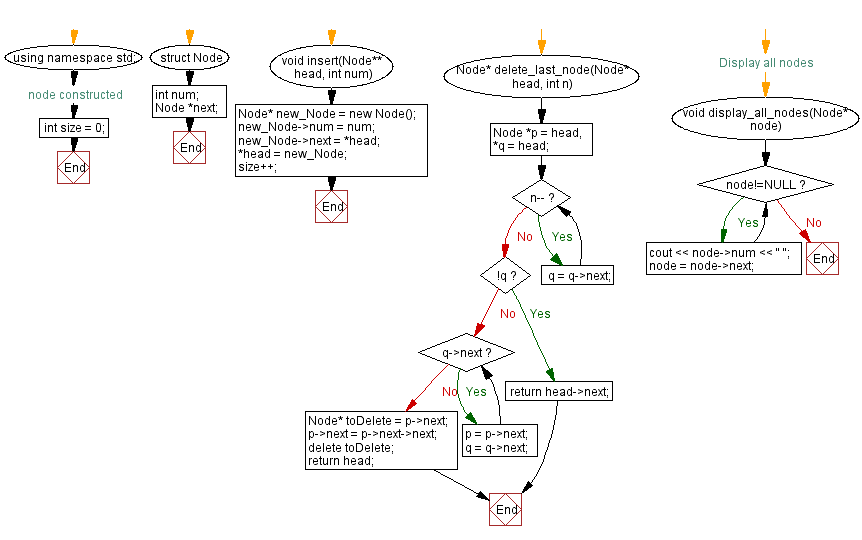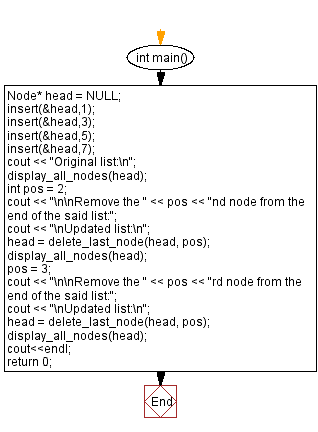﻿ C++ - Delete the nth node of a Singly Linked List from end

# C++ Linked List Exercises: Delete the nth node of a Singly Linked List from end

## C++ Linked List: Exercise-13 with Solution

Write a C++ program to delete the nth node of a Singly Linked List from the end.

Test Data:
Original list:
7 5 3 1
Remove the 2nd node from the end of the said list:
Updated list:
7 5 1
Remove the 3rd node from the end of the said list:
Updated list:
5 1

Sample Solution:

C++ Code:

``````#include <iostream>

using namespace std;

struct  Node
{
int num;
Node *next;
}; //node constructed

int size = 0;
Node* new_Node = new Node();
new_Node->num = num;
size++;
}

Node* delete_last_node(Node* head, int n) {
while (n--) q = q->next;
while (q->next) {
p = p->next;
q = q->next;
}
Node* toDelete = p->next;
p->next = p->next->next;
delete toDelete;
}

//Display all nodes
void display_all_nodes(Node* node)
{
while(node!=NULL){
cout << node->num << " ";
node = node->next;
}
}

int main()
{
cout << "Original list:\n";
int pos = 2;
cout << "\n\nRemove the " << pos << "nd node from the end of the said list:";
cout << "\nUpdated list:\n";
pos = 3;
cout << "\n\nRemove the " << pos << "rd node from the end of the said list:";
cout << "\nUpdated list:\n";
cout<<endl;
return 0;
}
``````

Sample Output:

```Original list:
7 5 3 1

Remove the 2nd node from the end of the said list:
Updated list:
7 5 1

Remove the 3rd node from the end of the said list:
Updated list:
5 1
```

Flowchart:CPP Code Editor:

Previous C++ Exercise: Delete the last node of a Singly Linked List.
Next C++ Exercise: Kth node from the Middle towards Head of a Linked List.

What is the difficulty level of this exercise?

﻿

## C++ Programming: Tips of the Day

Why is there no std::stou?

The most pat answer would be that the C library has no corresponding "strtou", and the C++11 string functions are all just thinly veiled wrappers around the C library functions: The std::sto* functions mirror strto*, and the std::to_string functions use sprintf.

Ref: https://bit.ly/3wtz2qA

We are closing our Disqus commenting system for some maintenanace issues. You may write to us at reach[at]yahoo[dot]com or visit us at Facebook